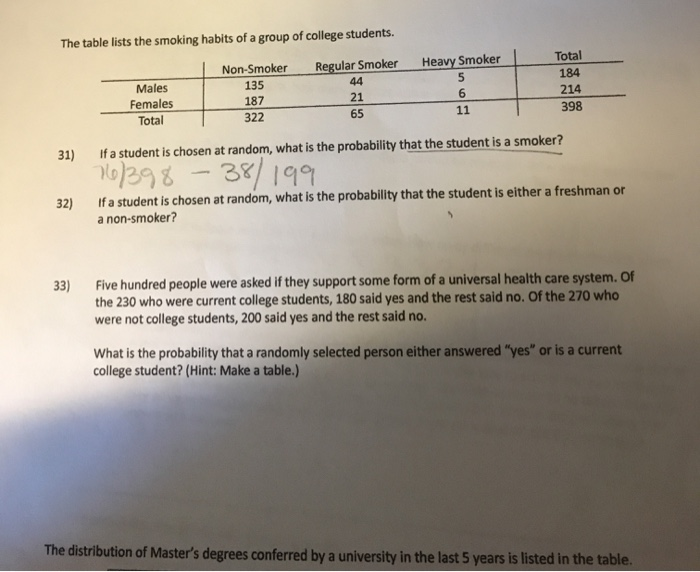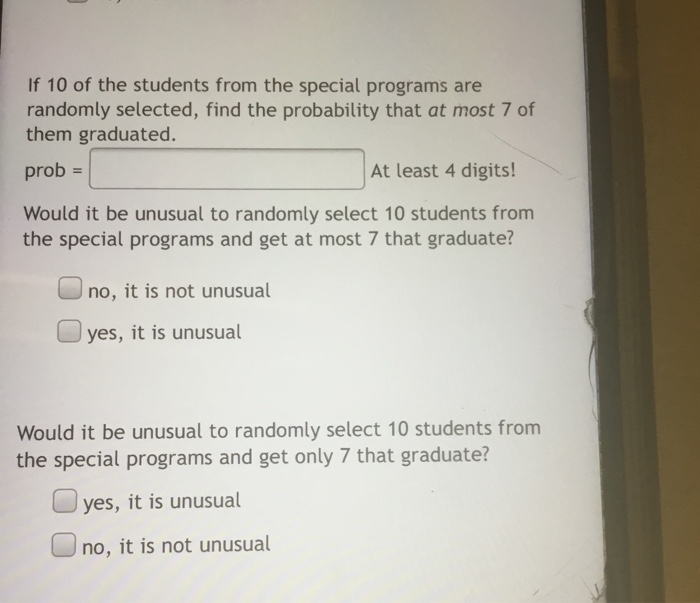# Poll of statistic students revealed that 44% study alone, 31% study in a group, 12% study both alone and in a group Find the probability that a randomly selected statistic student studies alone but not in a group

Poll of statistic students revealed that 44% study alone, 31% study in a group, 12% study both alone and in a group
Find the probability that a randomly selected statistic student studies alone but not in a group.

I made a Venn diagram, showing 12% in the intersection of the "alone" circle and the "group" circle.
It showed 32% falling in the "alone" only part, and 19% falling in the "group" only part.
This left 63% outside the two circles.
Does that mean that 63% don't study at all? Or they study in some other way?

Anyway the probability that they study alone and not in a group is .32
That was one of the answers it was a multiple choice question so I think you are right too Thanks
##### Add Answer of: Poll of statistic students revealed that 44% study alone, 31% study in a group, 12% study both alone and in a group Find the probability that a randomly selected statistic student studies alone but not in a group
Similar Homework Help Questions
• ### In a group of 100 students, 60 are taking math and 12 are taking English, but no one is taking both

In a group of 100 students, 60 are taking math and 12 are taking English, but no one is taking both. What is the probability that a randomly selected student from this group is taking either math or English?

• ### Find the test statistic for the following proportion: A collection of 500 randomly selected teachers revealed that 61% felt that all students should be required to take algebra in high school

Find the test statistic for the following proportion: A collection of 500 randomly selected teachers revealed that 61% felt that all students should be required to take algebra in high school.

• ### 3) Find the test statistic for the following proportion: A collection of 500 randomly selected teachers revealed that 61% felt that all students should be required to take algebra in high school

3) Find the test statistic for the following proportion: A collection of 500 randomly selected teachers revealed that 61% felt that all students should be required to take algebra in high school.

• ### In a group of 42 students, 22 take history, 17 take biology and 8 students took both history and biology. Find the proba...

In a group of 42 students, 22 take history, 17 take biology and 8 students took both history and biology. Find the probability of a randomly selected student a) Took both biology and statistics? b)Took biology, but not history? (In question a. my paper says statistics, it is not part of the given.)

• ### A group of 44 college students from a certain liberal arts college were randomly sampled and...

A group of 44 college students from a certain liberal arts college were randomly sampled and asked about the number of alcoholic drinks they have in a typical week. The purpose of this study was to compare the drinking habits of the students at the college to the drinking habits of college students in general. In particular, the dean of students, who initiated this study, would like to check whether the mean number of alcoholic drinks that students at his...

• ### If a person is randomly selected, find the probability that his or her birthday is not...

If a person is randomly selected, find the probability that his or her birthday is not in May. Ignore leap years. Group of answer choices a) 31/365 b) 31/334 c) 11/12 d) 334/365

• ### The table lists the smoking habits of a group of college students. Regular Smoker 44 Heavy...The table lists the smoking habits of a group of college students. Regular Smoker 44 Heavy Smoker Males Females Total Non-Smoker 135 187 322 Total 184 214 398 11 31) If a student is chosen at random, what is the probability that the student is a smoker? 107398 - 38/199 If a student is chosen at random, what is the probability that the student is either a freshman or a non-smoker? 32) 33) Five hundred people were asked if they...

• ### If 10 of the students from the special programs are randomly selected, find the probability that...If 10 of the students from the special programs are randomly selected, find the probability that at most 7 of them graduated. prob = At least 4 digits! Would it be unusual to randomly select 10 students from the special programs and get at most 7 that graduate? no, it is not unusual yes, it is unusual Would it be unusual to randomly select 10 students from the special programs and get only 7 that graduate? yes, it is unusual...

• ### 7% of all Americans live in poverty. If 44 Americans are randomly selected, find the probability...

7% of all Americans live in poverty. If 44 Americans are randomly selected, find the probability that d. Between 1 and 9 (including 1 and 9) of them live in poverty.

• ### suppose that 50% of all college students smoke cigarettes. a sample of 12 is selected randomly....

suppose that 50% of all college students smoke cigarettes. a sample of 12 is selected randomly. what is the probability that between 6 and 10 (both inclusive) students smoke? round your answer to four decimal places.

Free Homework App